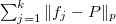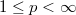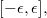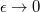﻿

### Best Simultaneous$L^p$ Approximation in the "Sum" Norm

#### Abstract

In this paper we consider best simultaneous approximation byalgebraic polynomials respect to the norm$\sum_{j=1}^k\|f_j-P\|_p$,$1\le p<\infty$. We prove an interpolation propertyof the best simultaneous approximations and we study the structureof the set of cluster points of the best simultaneousapproximations on the interval$[-\epsilon,\epsilon],$ as$\epsilon \to 0$.

DOI Code: 10.1285/i15900932v28n2p153

Keywords: Simultaneous approximation; Algebraic polynomials; Lp-Norm

Classification: 41A28; 41A10

Full Text: PDF